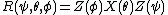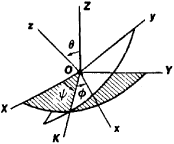# Euler Angles

(redirected from Euler angle)

## Euler angles

Three angular parameters that specify the orientation of a body with respect to reference axes. They are used for describing rotating systems such as gyroscopes, tops, molecules, and nonspherical nuclei. They are not symmetrical in the three angles but are simpler to use than other rotational parameters.

Unfortunately, different definitions of Euler's angles are used, and therefore it is confusing to compare equations in different references. The definition given here is the majority convention according to H. Margenau and G. Murphy.

Let OXYZ be a right-handed cartesian (right-angled) set of fixed coordinate axes and Oxyz a set attached to the rotating body (see illustration).

The orientation of Oxyz can be produced by three successive rotations about the fixed axes starting with Oxyz parallel to OXYZ. Rotate through (1) the angle ψ counterclockwise about OZ, (2) the angle Θ counterclockwise about OX, and (3) the angle &phgr; counterclockwise about OZ again. The line of intersection OK of the xy and XY planes is called the line of nodes.

Denote a rotation about OZ, for example, by Z (angle). Then the complete rotation is, symbolically, given by the equation below where the rightmost operation is donefirst.

## Euler Angles

(or Eulerian angles), the angles φ, θ, and ψ that define the position of a rectangular Cartesian coordinate system OXYZ with respect to another rectangular Cartesian coordinate system Oxyz of the same orientation (see Figure 1).Figure 1

Let OK be an axis, called the line of nodes, that coincides with the line of intersection of the coordinate plane Oxy of the first system with the coordinate plane OXY of the second system and is directed so that the axes Oz, OZ, and OK form a trihedral of the same orientation. The Euler angle φ, or angle of spin, is the angle between the axes Ox and OK, which is measured in the plane Oxy from Ox in the direction of the shortest rotation from Ox to Oy. The Euler angle θ, or angle of nutation, is the angle between Oz and OZ; it does not exceed π. The Euler angle ψ, or angle of precession, is the angle between the axes OK and OX, which is measured in the plane OXY from Ok in the direction of the shortest rotation from OX to OY. When θ = 0 or π, the Euler angles are not defined.

The Euler angles were introduced by L. Euler in 1748 and are used extensively in the dynamics of solids—for example, in the theory of the gyroscope—and in celestial mechanics.

## Euler angles

[′ȯi·lər ‚aŋ·gəlz]
(mechanics)
Three angular parameters that specify the orientation of a body with respect to reference axes.
References in periodicals archive ?
Therefore it is important to provide a basis to the antenna pattern angle definitions and aeronautical platform Euler angle definitions as in Figures 2 and 3, respectively.
Since the end-effector of the machine usually has a rotating tool, it is not necessary to include Euler angle [phi] among the DOFs of the machine.
Quaternion requires less calculation time, provides reliable orientation estimation and maintains stability compared to Euler angles or rotation matrix representation.
Euler angles [psi], [theta], and [phi] describe an orientation of frame B achieved by the sequential rotations, from alignment with frame A, of [psi] around [z.
Khawandi explains that the tech behind it measures three things: the acceleration, rotation rate and Euler angles, which are used in flight dynamics.
The main information measured by EBSD are Euler angles [[phi].
Movements of thorax segment were expressed in extension, left rotation, and lateral tilt with respect to the pelvis segment, by calculating Euler angles between these two segments.
The main problem in direct application of standard sliding PID control strategy to rigid body attitude control is definition of position error in terms of Euler angles.
We calculated the relative Euler angles between the two orientation sensors by expressing the relative orientation of sensor 2 with respect to sensor 1, using equations provided by XSens .
Let [omega] denote the set of Euler angles ([alpha], [beta], [gamma]} that takes the principal-axis frame to the laboratory frame.
im] may be described in terms of three Euler angles [Theta], [Phi], and [Eta];

Site: Follow: Share:
Open / Close